## Multirange Ohmmeter: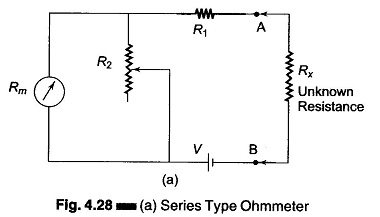The Multirange Ohmmeter circuit shown in Fig. 4.28 (a) is only for a single range of resistance measurement. To measure resistance over a wide range of values, we need to extend the ohmmeter ranges. This type of ohmmeter is called a multirange ohmmeter, shown in Fig. 4.29.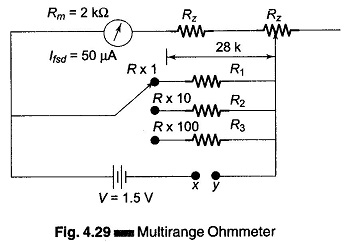### Shunt Type Ohmmeter:

The shunt type ohmmeter given in Fig. 4.30 consists of a battery in se­ries with an adjustable resistor R1, and a D’Arsonval movement.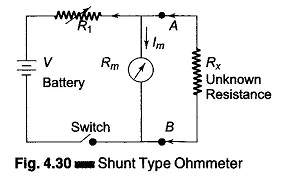The unknown resistance is con­nected in parallel with the meter, across the terminals A and B, hence the name shunt type ohmmeter.

### Calibration of the Shunt Type Ohmmeter:

To mark the “0” ohms reading on the scale, terminals A and B are shorted, i.e. the unknown resistance Rx = 0, and the current through the meter movement is zero, since it is bypassed by the short-circuit. This pointer position is marked as “0” ohms.

Similarly, to mark “∞” on the scale, the terminals A and B are opened, i.e. Rx = ∞ and full current flows through the meter movement; by appropriate selection of the value of R1, the pointer can be made to read full scale deflection current. This position of the pointer is marked “∞” ohms. Intermediate marking can be done by connecting known values of standard resistors to the terminals A and B.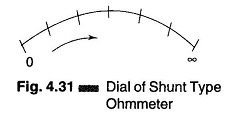This ohmmeter therefore has a zero mark at the left side of the scale and an ∞ mark at the right side of the scale, corresponding to full scale deflection current as shown in Fig. 4.31.

The shunt type ohmmeter is particularly suited to the measurement of low values of resistance. Hence it is used as a test instrument in the laboratory for special low resistance applications.

### Calibration of DC Instrument:

The process of calibration involves the comparison of a given instrument with a standard instrument, to determine its accuracy. A dc voltmeter may be cali­brated with a standard, or by comparison with a potentiometer. The circuit in Fig. 4.32 is used to calibrate a dc voltmeter; where a test voltmeter reading V is compared to the voltage drop across R. The voltage drop across R is accu­rately measured with the help of a standard meter. A rheostat, shown in Fig. 4.32, is used to limit the current.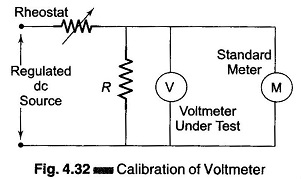A voltmeter tested with this method can be calibrated with an accuracy of ± 0.01%.

Calibration of Ohmmeter:

An ohmmeter is generally considered to be an instrument of moderate accuracy and low precision. A rough calibration may be done by measuring a stand and resistance and noting the readings on the ohmmeter. Doing this for several points on the ohmmeter scale and on several ranges allows one to obtain an indication of the accuracy of the instrument.

Scroll to Top